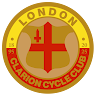Search
•London Clarion Cycle Club

# Welcome to the London (=C=L=A=R=I=O=N<) Cyclist!

This blog will include details of recent London (=C=L=A=R=I=O=N<) rides together with ramblings about Cycling in London and some interesting facts about the history of the (=C=L=A=R=I=O=N<) movement.